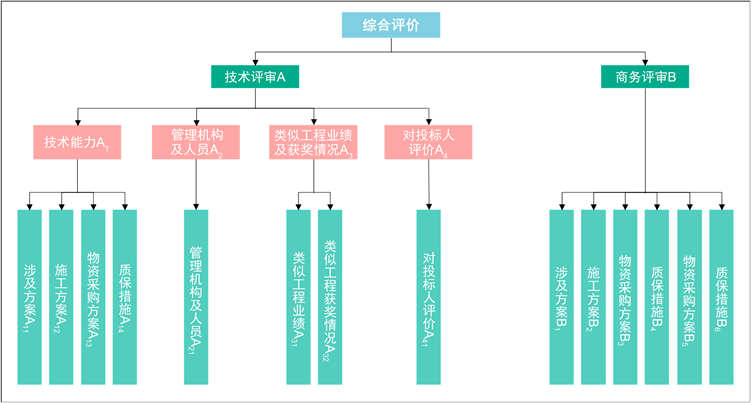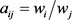﻿ 电网工程服务类采购评审体系优化方法研究

电网工程服务类采购评审体系优化方法研究Research on Optimization Method of Procurement Evaluation System of Power Grid Engineering Services

Abstract: From the perspective of the management and implementation of bidding activities of power grid enterprises, compared with the tendering and procurement of cargo services, it is difficult to com-prehensively, objectively and clearly reflect the evaluation objects in terms of covering procure-ment categories, describing demand characteristics, and selecting evaluation indicators. And the procurement needs of the project unit. In view of the above problems, this paper uses the analytic hierarchy process in the bidding process of the power grid, combined with the expert comprehen-sive evaluation method, according to the review purpose of the grid engineering service procure-ment project and the setting of the review elements, starting from the two major modules of tech-nology and business, with reference to the evaluation The index design principle selects the review index, and gives reasonable suggestions and directions, so as to establish an optimization method for the engineering service review index system. This article uses EPC general contract project bidding detailed technical review template and business review template as examples to propose optimization suggestions for the template review elements and review content settings, which can establish hierarchical structure model based on different project index systems, describing the specific optimization procedures of the analytic hierarchy process and comprehensive evaluation method to quantify the review elements. It is of great significance to ensure the progress and qual-ity of power grid construction by studying the evaluation system of procurement tenders for service procurement.

1. 引言

2. 评审模板应用现状分析及问题汇总

2.1. 评审模板未能全面覆盖所有采购品类

2.2. 部分模板评审内容全面性不足

2.3. 部分模板分值权重分配不合理

2.4. 部分模板的评审项重要性和可评性不足

1) 通过对商务和技术评审模板评审项的重要性和可评性评价的反馈信息进行比较，反映出重要性与可评性两大评价标准存在正相关的规律，即：重要性高的评审项，其可评性也相对较高。同时，包括商务评审模板的评审项存在指标可评性较高的反馈，而技术评审模板的评审项存在指标重要性评价高于可评性，存在评估方法、分值权重等设定不合理的情况。

2) 施工类评审模板评审项的重要性和可评性的评价反馈结果均高于其它类型模板。

3) 商务评审模板中，对包括企业资质、安全和质量保障指标及文件响应情况等在内的评审项有较高的刚性需求，这类指标在评审过程中的重要程度较高。

4) 技术评审模板中，包括技术方案、管理机构人员、安全和质量保障及控制措施、业绩及获奖情况等在内的评审项的重要性较高，对应指标的可评性也较高。

5) 此外，实际评审过程中包括商务评审模板中的银行资质、后续服务与技术模板中的造价控制、设施设备等评审项的重要性与可评性相对较低，具体分值权重的设定须结合各类模板评审项的重要程度而定。

2.5. 部分模板评审内容语言描述不清晰

2.6. 模板评审内容对评审工作量的影响

3. 相关理论与方法

3.1. 招投标评审的相关理论

1) 向量优化理论

2) 效用理论

3.2. 聚类分析法

3.3. 层次分析法

1) 建立问题的递阶层次结构模型；

2) 构造判断矩阵；

3) 层次单排序及一致性检验；

4) 层次总排序及一致性检验。

3.4. 模糊综合评判法

4. EPC总包项目模板应用分析

4.1. 建立服务类采购项目招标评审指标体系Table 1. Provincial company non-material-EPC general contract template technical template and business template

4.2. 层次分析法

4.2.1. 建立递阶层次结构模型

EPC总包项目招标要素评审指标体系的层次结构模型可为四层，第一层为目标层，第二层为准则层，由技术评审A、商务评审B两个模块组成，不同类型的项目技术模块评分和商务模块评分的比重不同，每个模块下设若干评审指标，第三层为一级指标层，对技术与商务评审表的一级指标要素评分，不同项目评审表的一级评审指标不同；第四层为二级指标层，由图可知，技术评审模块包含一、二级指标，而商务评审模块一、二级评审指标可视为相同。除此以外，实际层次模型还包含针对各个二级指标关于评审细则的设定、分值的评定。综上所述，针对EPC总包技术和商务详评模板，建立的要素评审指标体系的递阶层次模型结构(见图1)。Figure 1. The overall hierarchical structure model of EPC general contract comprehensive evaluation method

4.2.2. 构建判断矩阵Table 2. Comparison of n-th order judgment matrix ATable 3. Comparison scale and its meaning${a}_{ji}=1/{a}_{ij}$

4.2.3. 层次单排序及一致性检验

$W={\left({w}_{1},{w}_{2},\cdots ,{w}_{n}\right)}^{T}$

${w}_{i}^{0}={\left(\underset{j=1}{\overset{n}{\prod }}{a}_{ij}\right)}^{1/n}$

$i=1,2,\cdots ,n$

${w}_{i}=\frac{{w}_{i}^{0}}{\underset{i=1}{\overset{n}{\sum }}{w}_{i}^{0}}$

$i=1,2,\cdots ,n$

${\lambda }_{\mathrm{max}}=\frac{1}{n}\underset{i=1}{\overset{n}{\sum }}\frac{\underset{j=1}{\overset{n}{\sum }}{a}_{ij}{w}_{i}}{{w}_{i}}$

$CI=\frac{{\lambda }_{\mathrm{max}}-n}{n-1}$

$CR=CI/RI$Table 4. Random consistency index of 1~9 order judgment matrix

$CR<0.01$ 时，可以认为判断矩阵具有满意的一致性。否则，就必须重新调整判断矩阵A中元素，直至判断矩阵具有满意的一致性为止。

4.2.4. 层次总排序计算及一致性检验

$\begin{array}{cccccc}层次B\层次A& {A}_{1}& {A}_{2}& ...& {A}_{m}& 层次\\ & {a}_{1}& {a}_{2}& ...& {a}_{m}& 总排序权值\\ {B}_{1}& {b}_{11}& {b}_{21}& ...& {b}_{1m}& \sum {a}_{j}{b}_{1j}\\ {B}_{2}& {b}_{21}& {b}_{22}& ...& {b}_{2m}& \sum {a}_{j}{b}_{2j}\\ ...& ...& ...& ...& ...& ...\\ {B}_{n}& {b}_{n1}& {b}_{n2}& ...& {b}_{nm}& \sum {a}_{j}{b}_{nj}\end{array}$

$CR=\frac{\underset{j=1}{\overset{m}{\sum }}{a}_{j}C{I}_{j}}{\underset{j=1}{\overset{m}{\sum }}{a}_{j}R{I}_{j}}$

$CR<0.01$ 时，可以认为层次总排序具有满意的一致性。层次总排序计算得到的是一位专家给出的各项评审指标的权重值，因此，某一项指标的综合权重值 ${W}_{j}$ 应是所有专家意见的算术平均值。其计算式如下：

${W}_{j}=\frac{\underset{i=1}{\overset{m}{\sum }}{w}_{ij}}{m}$

Wij——第i位专家对第j项指标的评价权重；

m——专家人数。

4.3. 综合评价法计算投标方案的综合优度值

${A}_{j}=\underset{i=1}{\overset{4}{\sum }}{w}_{1i}{S}_{1ij}$

${B}_{j}=\underset{i=1}{\overset{6}{\sum }}{w}_{2i}{S}_{2ij}$

${Y}_{j}={A}_{j}+{B}_{j}$

Aj——第j位专家对技术评审模块的优度值；

Bj——第j位专家对商务评审模块的优度值；

Yj——第j位专家对投标方案的综合优度值；

W1j——技术评审模块指标A21，A22，…，A28的综合权重；

W2j——商务评审模块指标B31，B32，…，B37的综合权重；

S1ij，S2ij——第j位专家分别对技术评审、商务评审模块指标A1i，B2i的绝对评价标度分值。

$E=\underset{j=1}{\overset{m}{\sum }}{E}_{j}/m$

$A=\underset{j=1}{\overset{m}{\sum }}{A}_{j}/m$

$B=\underset{j=1}{\overset{m}{\sum }}{B}_{j}/m$

A——技术评审模块的综合优度值；

B——商务评审模块的综合优度值；

M——专家人数。

$Y=A+B$

5. 结语

 郭永旗. 电网建设项目评标指标体系及其应用研究(含招标流程及各类型项目评审指标) [D]: [硕士学位论文]. 长春: 吉林大学, 2009.

 周涛. 货物评标办法中分值权重系数设置的探讨[J]. 科技信息, 2012(32): 285-285.

 王宁宁, 刘学峰. 基于模糊层次分析的电网工程设计评标方法研究[J]. 项目管理技术, 2016, 14(5): 104-104.

 杜栋, 庞庆华. 现代综合评价方法与案例精选[M]. 北京: 清华大学出版社, 2005.

 梁占堂. ZYNF公司EPC总承包项目对施工承包商的招标研究[D]: [硕士学位论文]. 西安: 西安建筑科技大学, 2014.

 汪健斌. 应用层次分析法进行水电工程项目招标评审[J]. 湖北水力发电, 2005(2): 7-9.

 董海鹏. 电力企业设备、材料评标模型研究[D]: [硕士学位论文]. 北京: 华北电力大学, 2004.

Top